Properties of Energy & Matter by Cranwell
 Nothing is Solid. Space is not Empty. Everything is Connected.

 Font Size A A A
 by James Clifford Cranwell 2/17/98 Part II ... The Flux

It is well known that all matter in the Universe is composed of atoms and sub-atomic particles, held together by four and only four fundamental forces. These four forces are: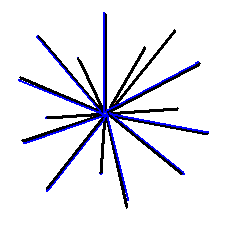The Strong force (short range) : 100 = 1 = Unit force

This is the force which powers the Sun. It is the devastating force behind hydrogen bombs and the force that binds protons / neutrons into nuclei. This unit force is given the value of one, or ten to the power of zero (100), all other forces are measured as a relative strength of it.

Electro-magnetic force (long range): 10-2

This is the force that binds atoms and molecules together and is also the force of electricity. It is the source of power behind for instance a light bulb. It is one hundredth the strength of Unit force so, the value is 1/100 or one divided by ten to the power of two = 1/(102) = 10-2

Weak force (short range): 10-9

This is the force responsible for radioactive decay and instability in certain nuclei. It is one billionth strength of Unit force so, its value is 1/1,000,000,000 or one divided by ten to the power of nine = 1/(109) = 10-9

Gravitational force (long range): 10-38

This is the force that holds the Earth in orbit around the Sun, keeps our feet firmly anchored to the ground and makes apples fall from trees. It is 1/(1038) (one divided by one followed by 38 zeroes) 1/100,000,000,000,000,000,000,000,000,000,000,000,000 strength of Unit force so, the value is one divided by ten to the power of 38 = 1/(1038) = 10-38

The Gravitational force between any two objects for example the Earth and Moon can be measured using one of Newton's equations F = Gm1m2/r 2 . The constant G in this instance is the Universal gravitational constant. Meaning the force of gravity equals the product of any two masses divided by their radius (separation) squared, quantity times G.
The value of the Gravitational constant is 6.67259 E-11 ...m3 kg-1 s-2
The exact value for this is G = 1/(10 * √26 -1)c ...m3 kg-1 s-2
G = 1/(10 * √26 - 1)c = 6.6725903807971 E-11 m3 kg-1 s-2 Exactor
In this instance the speed of light "c" is just an amount of meters or a specific number , not meters per second.
Which gives us the very intriguing...perfectly repeating...continued fractional form ...
1/(10 * √26 - 1)c= 1 / (10 [5,10,10,10,10,10,10,10,10,10,10,...10 ]-1)c
that fits in really nice in our ten dimensional Universe.
Square root 26 = [5,10,10,10,10,10,10,10,10,10,10,...10 ]
that's 5 and 1/10 with another 1/10 tacked onto the ten in the denominators forever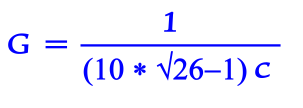```         1
5 + ----------------
1
10 + --------------
10 + 1/(10 + 1/(10 + 1/10... etc.))
```

#### G = 1/((10 * (√26))-1)c#### Flunot... center of axis (cut away view)

So, this is the exact value for the Universal Gravitational Constant. But more importantly this defines the exact shape of the Unit Flux. It's a particle and by simply altering its shape it becomes anything and everything in this Universe. It has 10 hypotenuse or diagonals joined at their centers, terminating on the vertices of the dodecahedron.
This is the same as twenty radii emanating from a common center called a Flunot...

Arrangement of Axis Unit Flux 10-D

It has ten diagonals (the normal state). They are composed of one dimensional (1-D) infinitesimal width string like objects arranged in a ten dimensional Dodecahedron axes pattern terminating on vertices or the set of twenty points...```    (+-x/y,  +-xy,     0)  where y = (√5+1)/2
( +-xy,     0, +-x/y)        x = G /(20 * √3)
(    0, +-x/y,  +-xy)        G = 1/(10 * √26 - 1)c
(  +-x,   +-x,   +-x)

y = (√5+1)/2 = 1.61803398875 = the golden ratio.
```
but, since we are at the quantum level where dimension is viable direction only, that makes ten dimensions in the actual space time continuum. The strings are only one dimension but are arranged in ten dimension Space. So the 10 different axes (20 different directions from origin) occupy or commandeer space in 10 dimensions without actually filling its volumetric area. Thereby leaving it almost empty.
The Flux particles set up their own field (face centred cubic spherical space type pack) relative to the object of largest mass in proximity. This corresponds to a lattice which the center of every sphere or particle is the set of all points (x,y,z) where x, y, and z are integers adding up to an even number (That's arrangement, not size).

Flux particle...Nine Dimensional dispersion or 10-9One axis is used to convey the force...either photonic or gravitational (that's vibrations and / or tension), the other Nine axes are one dimension each.
This is a ten dimensional Universe so, every axis is a power of ten. When a force or vibration is being transmitted through one axis of any particle it causes the 18 remaining radii repel away from the charge. As the shape changes from nine Dimension (remaining axes of dodecahedron) weak down to two dimension (disc) electron it causes the particle to separate from the field connections lattice along the way.
The number of field connections is the determinate factor of relative strength or dimension of any particle. Any amount of repel or dimension change is possible here allowing force values from 10-9 through 10-2 on any particle or field created.

Electron...Can disperse energy in 2-dimensions or 10-2 strengthWhen one axis of any particle meets up with a proton string and is held in place or a large force is being transmitted through it, two of the twenty radii are used as the Unit Force spike. The 18 remaining radii repel away from the charge into a two dimensional disc thus becoming the Electron.
This makes "18" the determinant number in Electron shell arrangements, check the larger noble gases: Argon, Krypton, Xenon, Radon, the amount of electrons in outermost shells will always sum to 18, the first three even have atomic numbers that are multiples of eighteen.
Three groups of six radii from one electron can form (along with seven other electrons) the corners of a cube or the "Octet Rule" (do the math...it works).

Electron

“We don’t know where the electron is?”

ummm… no, they don’t know what the electron is.

Think about all the things the electron is doing… storing different amounts of charge, absorbing and releasing different frequencies, leaving the atom and traveling at an exact speed (and the charge and frequency amounts go along with it), it has momentum, speed, spin?

How is all this possible if the electron is a zero point particle? A tiny sphere? Or cloud-like? It is not possible.

A tiny sphere can spin, move in a straight line or do nothing.
For a sphere to be a feasible model there would also have to be something that analyzes the rate of spin and releases and conveys the information.
How is a sphere going to store charge? It is not.
If this sphere is spinning… what is holding it in place? Are there tiny universal joints?

Electron Spherical model does not work

Nothing is possible at all with a cloud-like model.

An atom might have dozen of electrons. What is holding them in their orbitals? Think about it… the orbital paths are like concentric spheres, each one a little lager then previous.
What is holding the Electrons in their orbitals? Gravity? No… that works large scale if there is a planet or whatever and the size and speed are correct for the orbit.
If something like gravity is holding the electrons in place… how does it know the exact arrangements of the orbitals?
Is atomic orbital gravity computerized?

Something in there automatically configures to these specs…

Each s subshell holds at most 2 electrons
Each p subshell holds at most 6 electrons
Each d subshell holds at most 10 electrons
Each f subshell holds at most 14 electrons
Each g subshell holds at most 18 electrons

Something is really happening with the number 18…
http://en.wikipedia.org/wiki/Electron_shell

Clouds know about the number 18, correct?
I think tiny spheres know about the number 18 also…
if there is a group of 18 spheres and number 19 comes along… he is not allowed, maybe it was bad credit or something?

For all the Knuckleheads that are going to say… "maybe 18 is all that will fit…"
No, if the electrons are tiny spheres… thousands would fit in an orbital.

Figure it out… 18 is the magic number in there. Why?

p.s. How can you argue about something when you are not even sure if that something exists in the form you are thinking?

Proton...Zero Dimensions or Unit force strength...100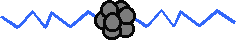A proton is 20 strings (like everything else) one radii string is attached to a neutron, one is attached to a electron and the other 18 remaining radii are balled up or collapsed.
If they collapse in groups of three each that would make 6 groups (6 * 3 = 18) or six types of Quarks in three flavors thus creating a proton with two string radii left. The flux strings always curl in groups of three (red, blue, green).
One Proton string and one Neutron string balled up or collapsed together is called a Meson.

If the proton were the size of the dot above the letter "i" then the strings could be compared to something a lot finer than the web of a spider extending out a few hundred meters. Fine enough where eighteen strings can curl into a space the size of the proton and have a spaghetti ball type configuration with a very loose string (or filament) pack.
It is the way to make the most universe with the least amount of material.
Newton's equation F = Gm1m2/r 2 breaks down and becomes ineffective at the proton - electron size or atomic realm, the particle shape has altered and the actual mechanism of attraction or attachment has changed.

Neutron... Nineteen curled radii, one spike left
(dying to curl up with a proton spike)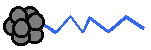The Neutron has one more string radius curl than the proton giving it slightly more mass. Protons can chain together as they curl, a Neutron is a nice way to dead-end the chain so the force of Gravity can ensue.
The string radii or spikes are the mechanism any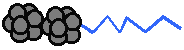particle... electron, proton or neutron etc. uses to connect and relay charge or vibration. In the atomic nucleus the last spike (one radius) of a neutron and one of the two proton spikes curl into a meson, that leaves the whole proton-neutron package with only one spike emanating from the proton, thereby leaving the neutron without an outlet, giving it a neutral charge.
This means an actual free neutron will have all twenty radii curled. If one curled neutron radius suddenly uncoils... it will convert into a proton with the ejecting charge or vibration creating an Electron in the process and is called Beta Radiation (actually one unit of string tension).
Note: if the curled radius in the proton / neutron connector opposite the proton spike actually breaks off from the proton, once again the neutron radius suddenly uncoils creating the electron and proton but this time there is a free radius or diameter also and is called a neutrino.

Working together as a group

Everything is made from strings. The basic particle is 10 strings joined at their centers (or 20 radii). When they are balled up they are a proton or neutron. The individual radii (1 of 20 strings) are the connectors used to connect neutron to protons (balled up) and proton to electrons (full length but twisted together). (more info on formations here)

An electron is shaped like the metal spines of an umbrella (without the hinges or fabric of course). One string extents from where your hand would hold it up to the center of axis. There, eighteen strings (or radii) extent out in the same type shape as the umbrella. The last string goes straight up (the same length as all the rest) and connects with the field in space (space is made of the same stuff by the way). This same basic particle (with nothing balled up or twisted) is also the strings used in free space for conveyance of light and gravity).

`(N)-(P)— — — — — — — — — — — — - E — — — — — — — — — — —`

Mass... for instance something that has a proton, is a particle with strings balled up. It doesn’t actually convert into energy... it releases what can be considered its energy by un-balling. The particle is still there but now its shape has changed into the regular particle (un-balled) 10 strings with common center (or 20 radii).

Everything Particle...

Remember Buckaroo's... everything is made out of particles.
That includes all forces and all fields.
A field is a group of particles.
A force is a particle(s) action on other particles (i.e. particles pulling other particles).
If anyone explains for example the four forces... what they are really saying is there are four different particles and / or groups of those particles. (actually it's the same particle doing four different things)

Electromagnetic field...? That would be a group of particles.
Gravitational field.....? That would be a group of particles.
Gravitational force.....? That would be a group of particles pulling.
The strong nuclear force...? That is supposedly a gluon -- a particle.
Everything (except energy) is either a particle or a group of particles.
(energy is vibrations traveling in particles (a photon is also only a particle vibration))

If you find forces or fields mentioned anywhere... just (mentally) replace the words "force" (or field) with "particle(s)." Then it's easy to see if the explanation is ridiculous because most times it becomes nonsense, can only work by magic or is just plain stupid.

Particles in explanations can also become doubled up... they might say a field (actually a group of particles) has a "mediator particle" or a "force carrier" (a particle). That is a great example of not knowing how something works so they add more stuff (actually the same stuff) in to try and explain it.

Everything is inert...
The energy contained in the nucleus of an atom is actually only potential energy.

The proton... that's a particle with most of it's strings balled up, sitting there completely inert with the field strings (by way of the electron strings) pulling tension on it / them from multiple directions.
When something like a reaction happens and the balled up proton strings can unwind... the field strings will pull (actually snap) all of the proton strings to their full length (that’s the same length as the field strings) going from the size of a proton to commandeering the volumetric size an atom takes up in space. This instantly creates the massive disruption (instant extra field particle strings) in the particle field in one spot and the electro-magnetic pulse.

Note: this is not the same as accelerating protons in the LHC. There, the protons actually do have energy because they are moving and smashing together.

Dark Energy -- Everything is pulled on equally from all directions by the field.
Gravity -- When 2 masses are introduced into the field they form endpoints and pull together.
Dark Matter -- That's the field itself... it is made from the same particles as everything else, it has mass but can't be seen (it is what is used to convey light and pull objects together).

In a proton all the strings are there but they are balled up. So when they unball and commandeer the size of an atom it was only a shape change.
I just found something else quite amazing because of this…
http://en.wikipedia.org/wiki/Atom
The width of an atom is 100,000 times larger than the nucleus.
That makes the volumetric (spherical) difference of helium one quadrillion… 1,000,000,000,000,000

That means one quadrillion nucleus sized spheres can fit inside a atom sized sphere.
So if a proton converts from balled up to atom size, that is the exact change in size of the field at the spot. The amount of disruption or change in field size (commandeering) is from nucleus size to atom size.
This is the equivalent of energy (vibrations into the field) released by the shape change.
It would be like having one particle then having one quadrillion particles.

The check… E = mc^2 …mass times speed of light is the amount.
And since everything is strings, and there are 20 string per particle, and there were 4 protons / neutrons in the nucleus (of this model).
Here is the answer (in meters per second)…

speed of light squared / one quadrillion = 89.8755179

89,875,517,900,000,000 m^2 / s^2
-----------------------
1,000,000,000,000,000

And there are 20 strings per particle…
89.8755179 / 20 = 4.49377589

And there where 4 particles (proton, neutron) in the nucleus…
4.49377589 / 4 = 1.12344397

Since the answer is almost exactly one… it means if you divided the "speed of light squared" up into one quadrillion proton sized particles with 20 strings each they would almost exactly fit inside an atom sized sphere.

You have to understand what I mean by commandeering to understand this.
p.s. if you take sphere packing into account the answer might be even closer to one. That's really freaky. You have to remember the 100,000 (thousand) sized difference (although very accurate) is only an estimate.

Mass (a proton) changes in shape and that shape change vibrates the field… that is energy. The proton was made of string and they are still there.
It is kind of like if you had a guitar string with tension on it and added a millimeter instantly to the length… it would make the string vibrate.
They call it potential energy.

Electric and Magnetic field

Both of those fields are only an effect that originates from the same thing… the actual field the encompasses space made of strings.
The electric and magnetic are just vibrations or a shape change in the real (flux) particle field.
An easy 2-D example is the highly stretched tennis net made of the finest spiders web.
If you were on one side of the net and plucked a string, the vibration would travel along string to the other side of the net. That vibration would set off perpendicular vibrations (and in the real field a shape change if strong enough) up and down in it’s travels.

If you ask… "wouldn’t the whole net just vibrate?" Yes, of course it would but using another example of an actual spiders web…
If the spider is sitting directly in the middle of his web and something (a bug) lands in his web… he knows the exact direction and placement of the bug.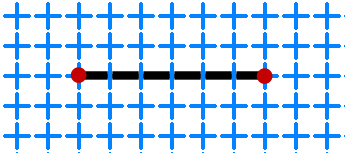This is because the spider and the bug have now actually set up endpoints for a more powerful string vibration… there is a mass on both ends of the web string. And of course whatever string had the initial pluck would have the most powerful vibration.
Remember this is a linear one string example in a real field the vibration would expand away spherically. But there is of course always endpoints, every atom with protons / neutrons is an endpoint.

"Spooky action at a distance...?" that’s when two endpoints set up their own string connection.

The Intrepid Photon?

What they refer to as a photon is actually just a vibration traveling along the strings. (not the string theory type)
That is why Wave Particle Duality is a mess...
Light is not a particle. That would mean there is some type of matter involved.

If light were a particle it would be some type of matter.

Does anyone really think there is a "matter stream" leaving your flashlight at the speed of light when you turn it on?. Think about how much matter would be bombarding us from the Sun... where is it all going?
Visible Light is only a small section of the electromagnetic spectrum...
There is a huge amount of other frequencies.
And if they are all "matter streams" it would mean that space is filled with vibrating matter (particles) coming and going (zipping around at the speed of light) in every possible direction, frequency and intensity.

Think about how many particle collisions there would be.

A photon is a particle...? Nope... that's ridiculous, and even though it's wrong it still results in space being filled with particles (which is actually correct... they are just not zipping around).

Quantum Weirdness:

Electrical energy is used to power mechanical devices but at the quantum level Electrical energy is just a frictionless mechanical vibration.

 Properties of Energy & Matter * part III *

Goddess 401 Home Page      admin@gootar.com     https://xulfrepus.neocities.org/